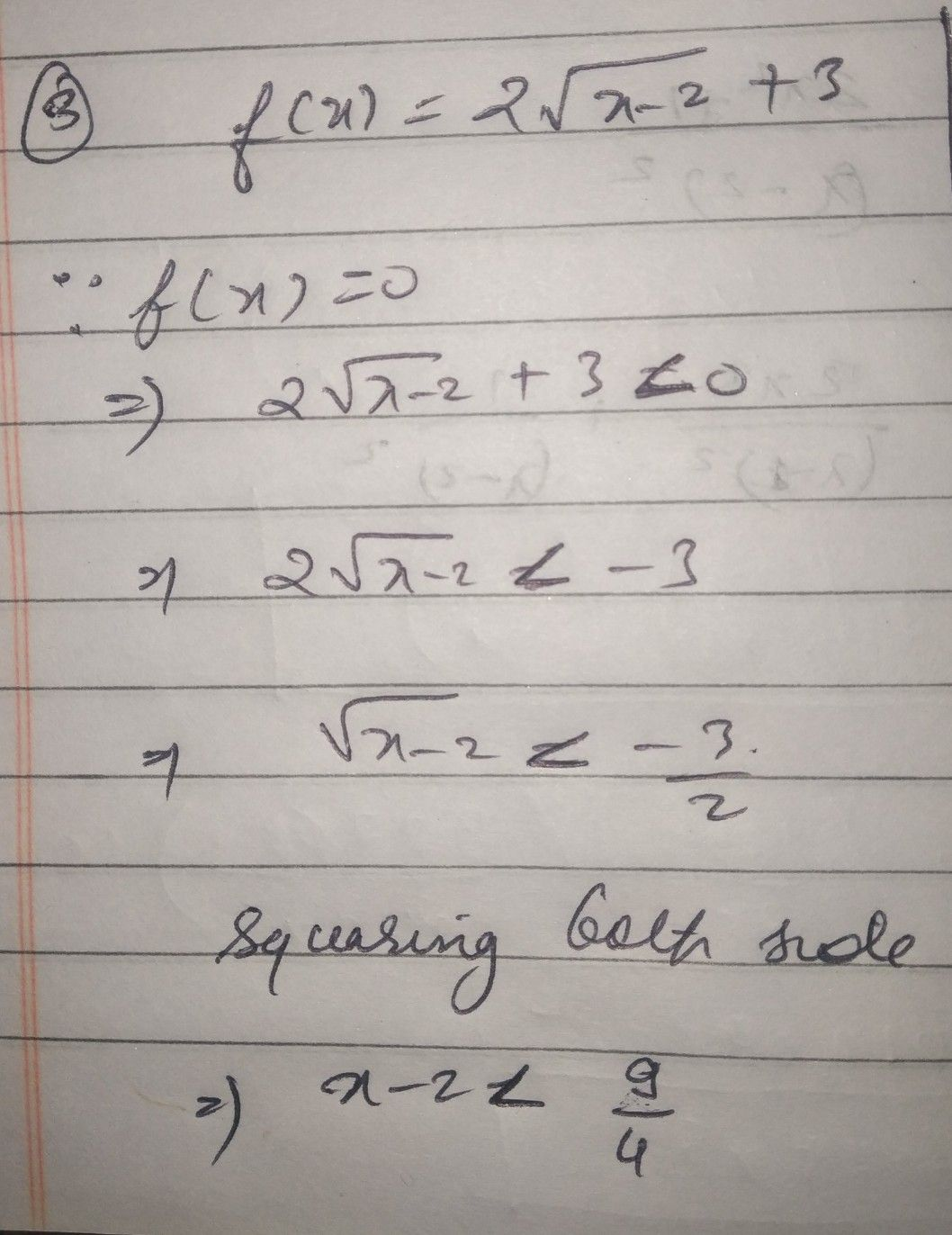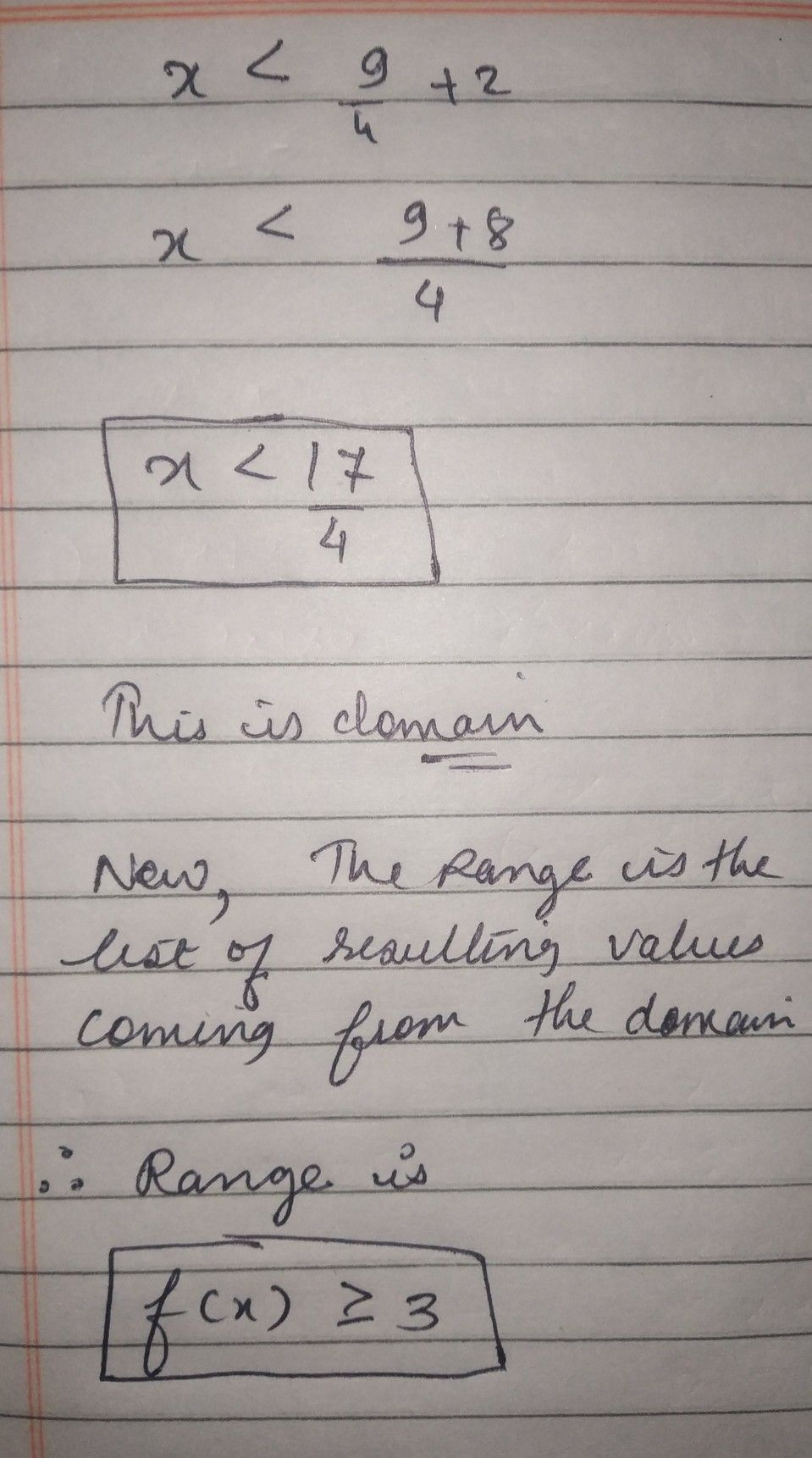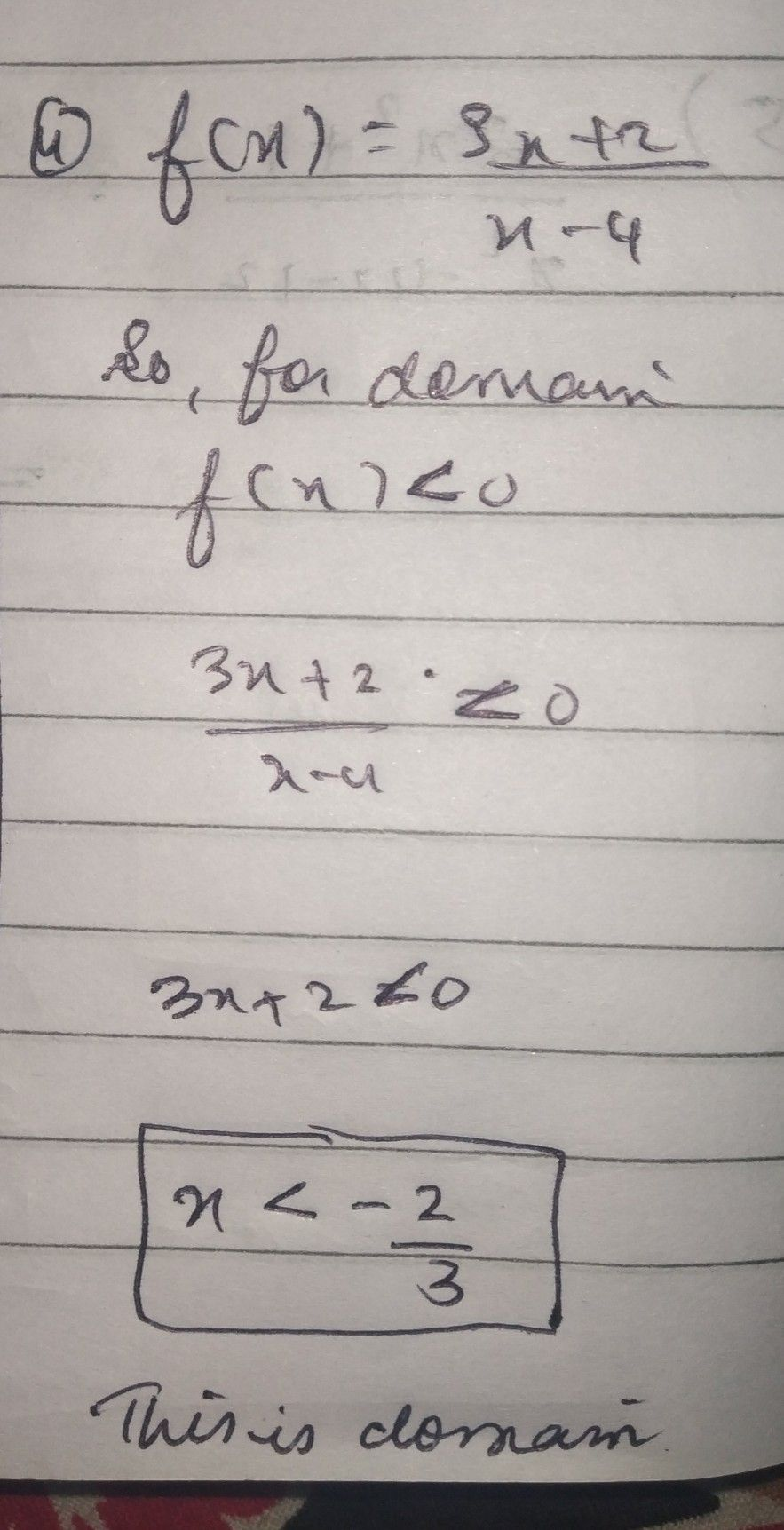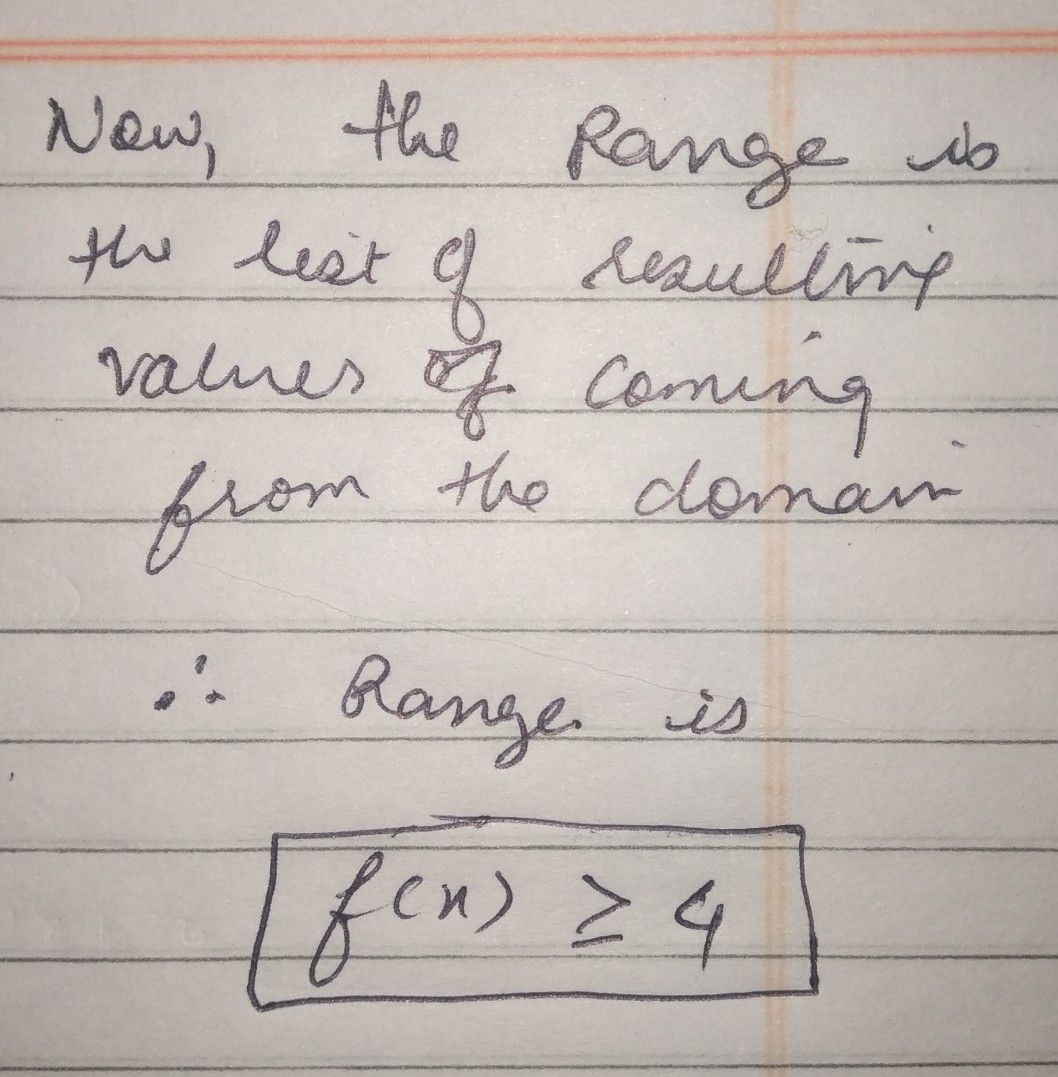Symbol
Problem$3$ What is the domain and range of the inverse function $f\left(x\right)=2\sqrt{x-2} +3$ $4$ Find the domain and range of the function inverse $f\left(x\right)=\dfrac {3x+2} {x-4}$
10th-13th grade
Other
Search count: 126
SolutionQanda teacher - mamta